# Introduction

There are three main forms of color-deficient vision, depending on which of the three types of cones (red, green, or blue) are missing or defective in the eyes of an individual.

1. Deuteranopes (about 6% of men) are red-blind, and so they have trouble distinguishing red from green.
2. Protanopes (about 2% of men) are green-blind, and they also have trouble distinguishing red from green.
3. Tritanopes (less than 1% of men) are blue-blind, and they have trouble distinguishing blue from yellow.

Polychrome includes facilities to simulate different forms of color deficit and to create palettes that can be used by individuals with color deficient vision. This vignette describes those facilities.

# Red-Green Color Maps

``````library(Polychrome)
``````

Next, we create the common “standard” color map used by bioinformaticians to draw heatmaps.

``````rg <- colorRampPalette(c("red", "black", "green"))(64)
image(matrix(1:64), col=rg)
``````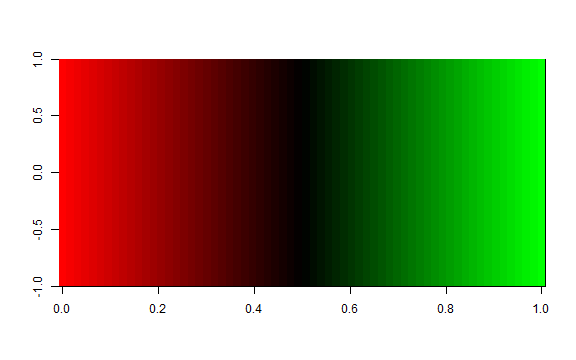Now, we convert it to what would be seen by a deuteranope.

``````rg.deut <- colorDeficit(rg, "deut")
image(matrix(1:64), col=rg.deut)
``````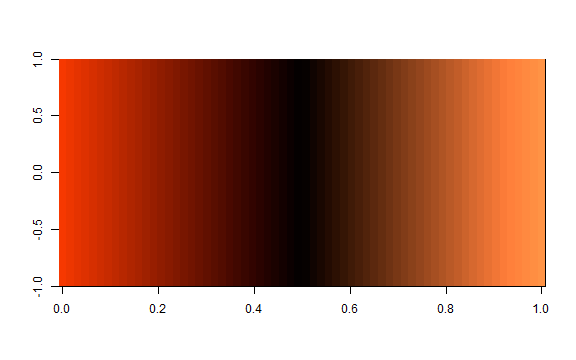We can also convert it to what would be seen by a protanope…

``````rg.prot <- colorDeficit(rg, "prot")
image(matrix(1:64), col=rg.prot)
``````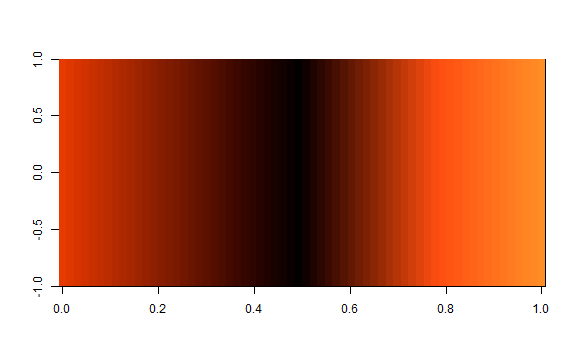… or a tritanope.

``````rg.trit <- colorDeficit(rg, "trit")
image(matrix(1:64), col=rg.trit)
``````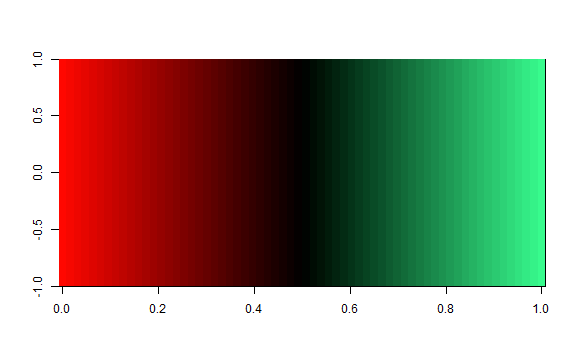# Large Qualitative Palettes

We repeat those operations for the 26-color alphabet palette from Polychrome.

``````alfa <- alphabet.colors(26)
swatch(alfa)
``````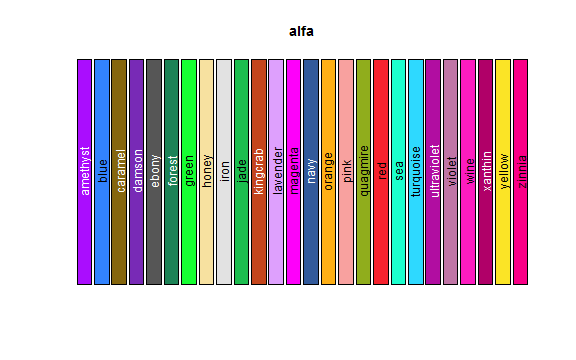``````alfa.deut <- colorDeficit(alfa, "deut")
swatch(alfa.deut)
``````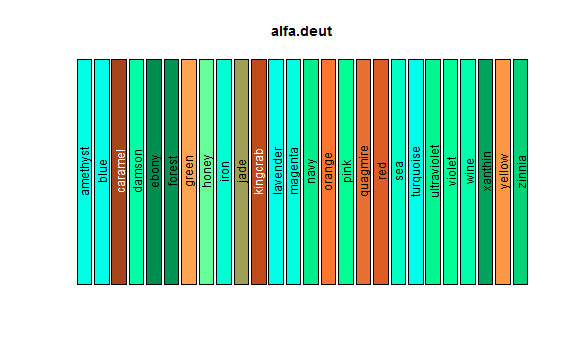``````alfa.prot <- colorDeficit(alfa, "prot")
swatch(alfa.prot)
``````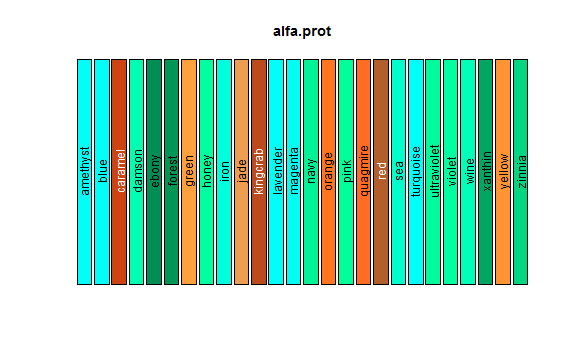``````alfa.trit <- colorDeficit(alfa, "trit")
swatch(alfa.trit)
``````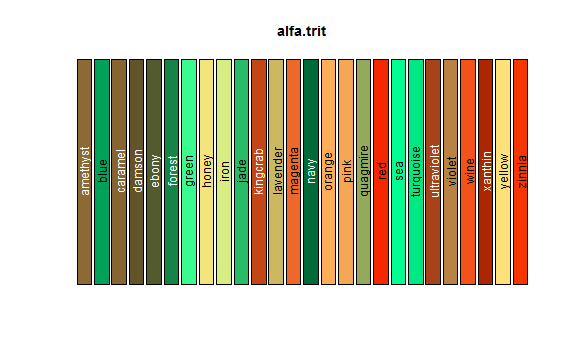# Creating New Palettes

We can also use the createPalette function to create new palettes designed for individuals with color deficits. This operation requires us to use the target option of the function. The default value of “normal” assumes that the target audience consists of individuals with normal color vision.

``````cp <- createPalette(20, "#111111", target="deuteranope")
names(cp) <- colorNames(cp)
swatch(cp)
``````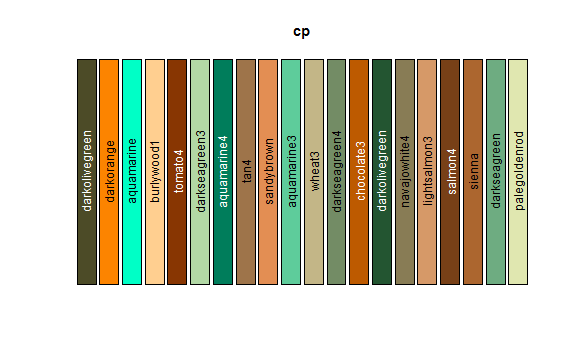``````cp <- createPalette(20, "#111111", target="protanope")
names(cp) <- colorNames(cp)
swatch(cp)
``````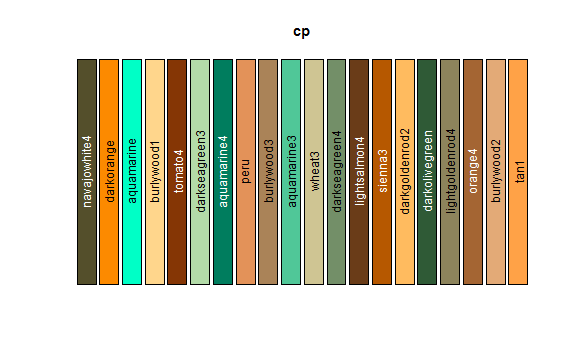``````cp <- createPalette(20, "#111111", target="tritanope")
names(cp) <- colorNames(cp)
swatch(cp)
``````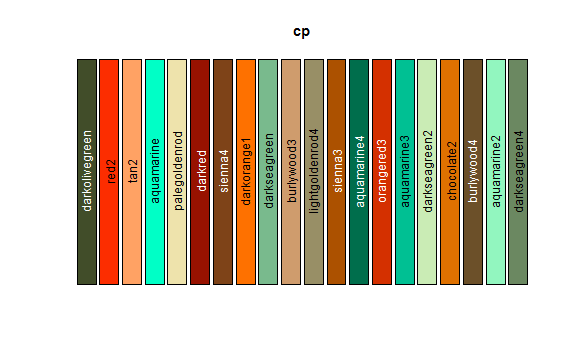## A Small Color-Safe Palette

Here we try to build a small palette that has at least some chance of being useful for people with any of the three forms of color-blindness. First, we get a slightly larger starting palette, and convert it to simulate each of the three forms of color deficits.

``````p34 <- palette36.colors(36)[3:36]
names(p34) <- colorNames(p34)
p34.deut <- colorDeficit(p34, "deut")
p34.prot <- colorDeficit(p34, "prot")
p34.trit <- colorDeficit(p34, "trit")
``````

Next, we reorder the palettes by distinguishability.

``````shift <- function(i, k=34) c(i, 1:(i-1), (1+i):k)
co <- shift(13)
pd <- computeDistances(p34.deut[co])
pp <- computeDistances(p34.prot[co])
pt <- computeDistances(p34.trit[co])
``````

Now we use the ranks from this ordering to compute a distinguishability score.

``````rd <- rank(pd)[order(names(pd))]
rp <- rank(pd)[order(names(pp))]
rt <- rank(pd)[order(names(pt))]
score <- 2*rd + 1.5*rp + rt
``````

Now we show the top ten colors (ranked by this score) , adjusting for different color deficits.

``````x <- p34[names(rev(sort(score)))][1:10]
y <- colorDeficit(x, "deut")
z <- colorDeficit(x, "prot")
w <- colorDeficit(x, "trit")

opar <- par(mfrow=c(2,2))
swatch(x, main="Normal")
swatch(y, main="Deuteranope")
swatch(z, main="Protanope")
swatch(w, main="Tritanope")
``````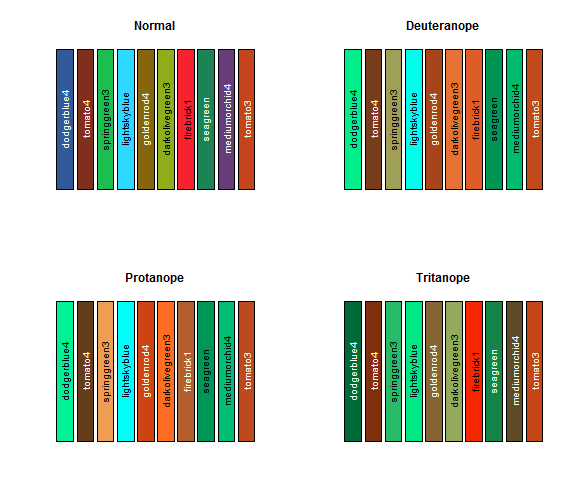# Conclusion

We have illustrated how to convert arbitrary palettes into versions that simulate different forms of color deficits. We have also shown how to create palettes specifically for individuals with different color deficits. Finally, we have created a ten-color palette that has a reasonable chance of working simultaneously for individuals with any of the three most common form of color deficits.